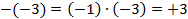Assumptions of Algebra

INTRODUCTION

The word “algebra” came to Europe from the Arabic word “al-jabr” from a book by the Persian mathematician Muhammad ibn Musa al-Khwarizmi written in about 830 A.D. and titled “Addtion and Subtraction after the Method of the Indians”.   Al-Khwarizmi’s family apparently practiced the old Zoroastrian religion.   But this was simply a rehash of earlier developments in Mesopotamia, Egypt, and Greece.   One of the earliest texts describing the algebraic method was the “Rhind Papyrus” written about 1650 B.C. by the Egyptian Ahmes, who transcribed it from an earlier work that he dated to between 2000 and 1800 B.C.   The Greek mathematician Iambichus in his book “Introductio Arithmatica” mentions an earlier work by Thymaridas (400 B.C. to 350 B.C) on the algebra of solving simultaneous linear equations.

In any event, in modern times the fundamental axioms for addition and multiplication are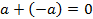Inverse Law of Addition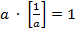Commutative Law of Addition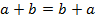Associative Law of Addition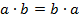Inverse Law of Multiplication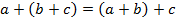Commutative Law of Multiplication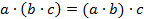Associative Law of Multiplication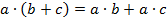Distributive Law

DIVISION AND SUBTRACTION

Unlike the operations of addition and multiplication, subtraction and division are not so well behaved.

For instance, we define the subtraction operator to be to change the sign of the second argument “b” and then to add, as followsThe following equation is just barely defined because the subtraction operation in this case is assumed to progress from left to right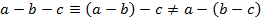For the operation of division, we define the operator to be to invert the second argument “b” and then to multiply, as follows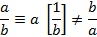And again we proceed from left to right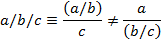MULTIPLICATION OF INTEGERS

If we have the positive integers “a”, “b”, and “c” such that a>0 and b>0 and c>0.

Then we can write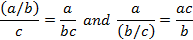or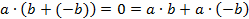So the multiplication of a positive and a negative is a negative number.

And also in a similar manner we can write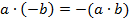or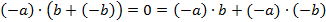And so the multiplication of two negatives is a positive number.

MINUS SIGNS

Unfortunately there is often some misunderstanding concerning the dreaded minus sign “-“.   This can have one of roughly three separate meanings as follows

1.      The minus sign, when applied to a number, is simply part of the name.   Some examples might be “-3” or “-17.2”.  In this case the minus sign simply designates a specific number which happens to be negative.  Note that positive numbers such as “+2” often are written without the leading plus sign “+”.

2.      When applied to a variable or an expression, it is short hand for multiplication by “-1”.  So for example we might have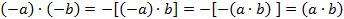or in an expression as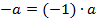3.      When used as an operator between two quantities a minus sign means to subtract or